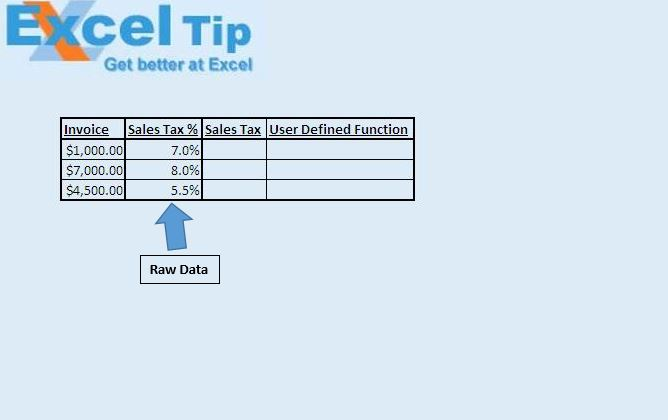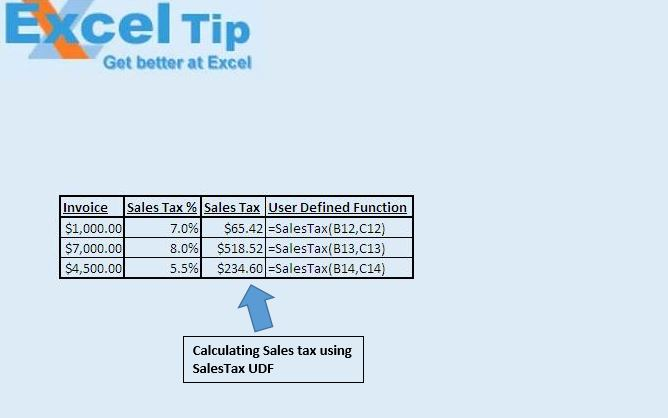# Calculating sales tax using VBA in Microsoft Excel

In this article, we will create a custom function to calculate sales tax for the given invoice and sales tax percentage.

Raw data for this example consists of invoice and sales tax percentage, we will calculate the amount of sales tax included in the amount in an invoice.We will use custom function “SalesTax” to get the amount of sales tax. This function takes invoice and sales tax percentage as input.Logic explanation

Below mathematical formula is used to calculate the amount of sales tax when invoice and sales tax percentage are present.

SalesTax = TotalInvoice - TotalInvoice / (1 + TaxPercentage)

We have used the above formula as it is in the “SalesTax” custom function.

```
Option Explicit

Function SalesTax(TotalInvoice As Double, TaxPercentage As Double)

'Formula used for finding the sales tax
SalesTax = TotalInvoice - TotalInvoice / (1 + TaxPercentage)

End Function

```